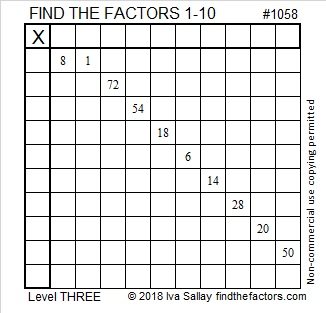# 1058 and Level 3

You can solve this puzzle! Just start with the easy clues closest to the top and work your way down cell by cell until you have the numbers from 1 to 10 in both the first column and the top row. Have fun!Print the puzzles or type the solution in this excel file: 10-factors-1054-1062

Now I’ll share some information about the number 1058:

• 1058 is a composite number.
• Prime factorization: 1058 = 2 × 23 × 23, which can be written 1058 = 2 × 23²
• The exponents in the prime factorization are 1 and 2. Adding one to each and multiplying we get (1 + 1)(2 + 1) = 2 × 3  = 6. Therefore 1058 has exactly 6 factors.
• Factors of 1058: 1, 2, 23, 46, 529, 1058
• Factor pairs: 1058 = 1 × 1058, 2 × 529, or 23 × 46
• Taking the factor pair with the largest square number factor, we get √1058 = (√529)(√2) = 23√2 ≈ 32.52691This site uses Akismet to reduce spam. Learn how your comment data is processed.